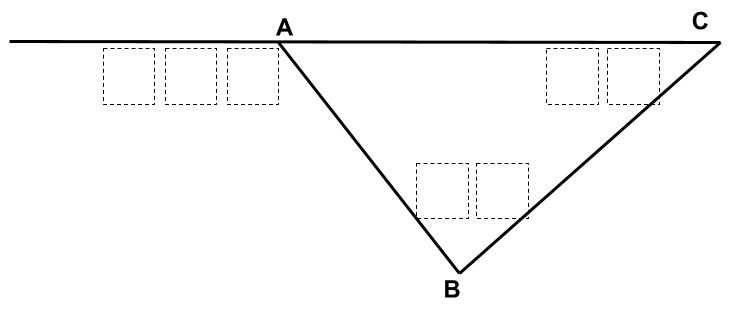# Interior and Exterior Angles of Triangles

Directions: In triangle ABC, angle ABC is obtuse. Using the digits 1 to 9 at most one time each, place a digit in each box to make angle ACB the smallest possible acute angle.### Hint

What does the first number in the three-digit number have to be?
What does the first number in the obtuse angle have to be?
What numbers do not work as the first digit of the acute angle? Why?

42 Degrees
138 = 96 + 42
137 = 95 + 42

Source: Jay Sydow

## Square Root Expression 2

Directions: Using the digits 0 to 9 at most one time each, place a digit …

1.A great extension of this problem would be to remove the restriction that angle ABC is obtuse and additionally pose slightly different questions:

What measures of angle ACB are possible?

What measure(s) of angle ACB yield multiple possible values of angle ABC?

2.Today, one of my students beat this. She found 37 degrees = angle ACB. The two remote interior angles would be 37 degrees & 89 degrees and would add to the exterior angle of 126 degrees.

•89 Degrees is not obtuse as required by the instructions